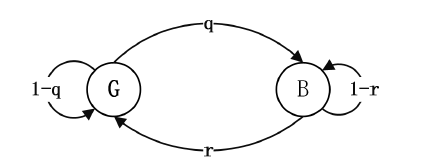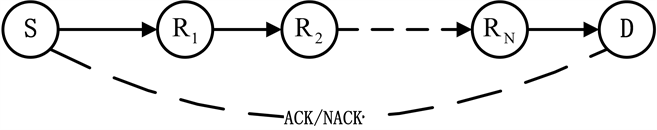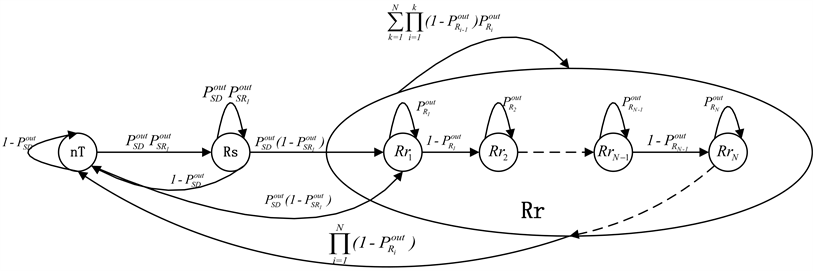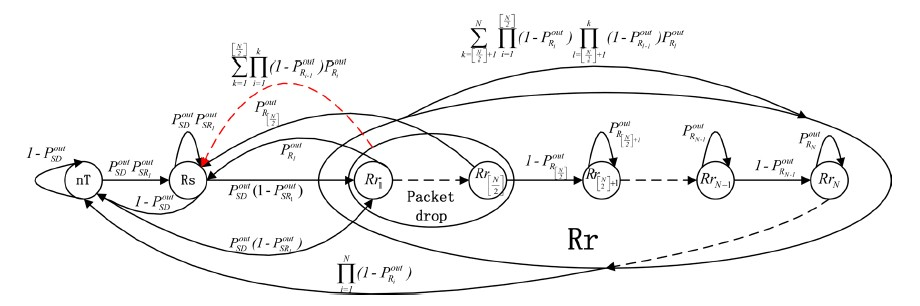# 记忆信道中基于三状态Markov链模型的多跳ARQ协议吞吐量的分析Throughput Analysis of Multi-Hop ARQ Based on Three-State Markov Model over Memory Channels

DOI: 10.12677/CSA.2018.87120, PDF, HTML, XML, 下载: 494  浏览: 4,686

Abstract: Multi-hop relaying is an effective technique to combat the negative impacts of wireless communication caused by channel fading, so it is widely used in wireless networks. Firstly, we introduced Ray-leigh fading channel and described the “memory” by using the two state Gilbert Elliott channel models, then obtained the state transition probability of the channel in stationary state. Secondly, we have established the three-state Markov chain model of the multi-hop ARQ (Automatic Repeat Request) system, and obtained the analytical solution for the throughput of multi-hop ARQ systems under the condition of the relay node without the packet loss. Finally, we considered the packet loss of relay nodes and set the critical relay for the packet loss to be [N/2]-th, and obtained the analytical solution for the throughput of multi-hop ARQ systems under the packet loss of relay nodes through the three-state Markov chain model of the multi-hop ARQ system. In fact, it can be concluded that the relay node without the packet loss has better throughput than the packet loss.

1. 引言

2. 记忆信道模型

$X\left(k\right)=\left\{\begin{array}{l}G\text{\hspace{0.17em}}\text{\hspace{0.17em}}\text{\hspace{0.17em}}\text{\hspace{0.17em}}\text{if}\text{\hspace{0.17em}}\gamma \left(k\right)>{\gamma }_{thr}\\ B\text{\hspace{0.17em}}\text{\hspace{0.17em}}\text{\hspace{0.17em}}\text{\hspace{0.17em}}\text{if}\text{\hspace{0.17em}}\gamma \left(k\right)\le {\gamma }_{thr}\end{array}$ (1)

$\left\{\begin{array}{l}P\left[X\left(k+1\right)=G|X\left(k\right)=G\right]=1-q\\ P\left[X\left(k+1\right)=B|X\left(k\right)=G\right]=q\\ P\left[X\left(k+1\right)=B|X\left(k\right)=B\right]=1-r\\ P\left[X\left(k+1\right)=G|X\left(k\right)=B\right]=r\end{array}$

$\left(\begin{array}{cc}1-q& q\\ r& 1-r\end{array}\right)$

${P}^{out}=\frac{q}{q+r}$ (2)

${P}^{out}=1-{\text{e}}^{b}$ (3)Figure 1. Gilbert Elliott channel

$q=\frac{1-{\text{e}}^{b}}{{\text{e}}^{b}}r$ (4)

$r=\frac{Q\left(\theta ,\rho \theta \right)-Q\left(\rho \theta ,\theta \right)}{{\text{e}}^{b}-1}$

$\theta =\sqrt{\frac{2b}{1-{\rho }^{2}}}$

$\rho ={J}_{0}\left(2\text{π}\cdot {f}_{D}{T}_{f}\right)$

${F}_{X,Y}=\frac{{\stackrel{¯}{\gamma }}_{X,Y}}{{\gamma }_{thr}}$ (5)

${\stackrel{¯}{\gamma }}_{X,Y}=\frac{{E}_{S}}{{N}_{0}}{\left(\frac{{d}_{X,Y}}{{d}_{0}}\right)}^{-\lambda }$

3. 多跳ARQ系统的三状态Markov链模型及系统吞吐量的分析

① 中继节点将数据包发送之后，仍保留有数据包的副本，即中继节点不丢包的情况；

② 中继节点将数据包发送之后，不再保留数据包，即中继节点丢包的情况。

3.1. 中继节点不丢包的三状态C-ARQ协议

① 节点S同时向目的节点D和第一个中继节点 ${R}_{1}$ 发送新的数据包。

② 如果中继正确接收了数据包，它会告知源节点。Figure 2. Multi-hop ARQ

③ 如果目的节点D正确接收了数据包，它会向源节点S和中继节点同时发送ACK应答，然后，源节点S又会进行一个新的数据包的传送。

④ 如果目的节点D和中继节点 ${R}_{1}$ 都没有正确接收数据包，源节点S将会重传这个数据包。

⑤ 如果目的节点D没有正确接收数据包，但中继 ${R}_{1}$ 正确接收了，目的节点D将发送NACK应答给源节点S，中继 ${R}_{1}$ 将承担发送任务，他会向中继 ${R}_{2}$ 发送此数据。

⑥ 如果 ${R}_{i}$ 没有正确接收来自 ${R}_{i-1}$ 的数据包，中继 ${R}_{i}$ 将会向 ${R}_{i-1}$ 发送NACK应答，同时 ${R}_{i-1}$${R}_{i}$ 重新发送此数据包。

⑦ 如果中继 ${R}_{i}$ 正确接收了数据包，它将承担起传送任务，然后将此数据包发送给中继 ${R}_{i+1}$

⑧ 重传过程往往会重复出现，直到传送的数据包被目的节点D正确接收。

${M}_{1}=\left[\begin{array}{ccc}1-{P}_{SD}^{out}& {P}_{SD}^{out}{P}_{S{R}_{1}}^{out}& {P}_{SD}^{out}\left(1-{P}_{S{R}_{1}}^{out}\right)\\ 1-{P}_{SD}^{out}& {P}_{SD}^{out}{P}_{S{R}_{1}}^{out}& {P}_{SD}^{out}\left(1-{P}_{S{R}_{1}}^{out}\right)\\ \underset{i=1}{\overset{N}{\prod }}\left(1-{P}_{{R}_{i}}^{out}\right)& 0& \underset{k=1}{\overset{N}{\sum }}\underset{i=1}{\overset{k}{\prod }}\left(1-{P}_{{R}_{i-1}}^{out}\right){P}_{{R}_{i}}^{out}\end{array}\right]$ (6)

$\left\{\begin{array}{l}\pi {M}_{1}=\pi \\ \underset{i=1}{\overset{3}{\sum }}{\pi }_{i}=1\end{array}$

${T}_{1}={\pi }_{1}=\frac{\left(1-{P}_{SD}^{out}{P}_{S{R}_{1}}^{out}\right)\underset{i=1}{\overset{N}{\prod }}\left(1-{P}_{{R}_{i}}^{out}\right)}{{P}_{SD}^{out}\left(1-{P}_{S{R}_{1}}^{out}\right)+\underset{i=1}{\overset{N}{\prod }}\left(1-{P}_{{R}_{i}}^{out}\right)}$ (7)Figure 3. Three-state Markov model for C-ARQ with no packet drop at the relay

3.2. 中继节点丢包的三状态C-ARQ协议

① 节点S同时向目的节点D和第一个中继节点 ${R}_{1}$ 发送新的数据包。

② 如果中继正确接收了数据包，它会告知源节点。

③ 如果目的节点D正确接收了数据包，它会向源节点S和中继节点同时发送ACK应答，然后，源节点S又会进行一个新的数据包的传送。

④ 如果目的节点D和中继节点 ${R}_{1}$ 都没有正确接收数据包，源节点S将会重传这个数据包。

⑤ 如果目的节点D没有正确接收数据包，但中继 ${R}_{1}$ 正确接收了，目的节点D将发送NACK应答给源节点S，中继 ${R}_{1}$ 将承担发送任务，他会向中继 ${R}_{2}$ 发送此数据。

⑥ 如果 ${R}_{i}$ 没有正确接收来自 ${R}_{i-1}$ 的数据包，当 $i>\left[\frac{N}{2}\right]+1$ 时，中继 ${R}_{i}$ 会向 ${R}_{i-1}$ 发送NACK应答，同时 ${R}_{i-1}$${R}_{i}$ 重新发送此数据包；否则，源节点S将会重传这个数据包。

⑦ 如果中继 ${R}_{i}$ 正确接收了数据包，它将承担起传送任务，然后将此数据包发送给中继 ${R}_{i+1}$

⑧ 重传过程往往会重复出现，直到传送的数据包被目的节点D正确接收。

${M}_{2}=\left[\begin{array}{ccc}1-{P}_{SD}^{out}& {P}_{SD}^{out}{P}_{S{R}_{1}}^{out}& {P}_{SD}^{out}\left(1-{P}_{S{R}_{1}}^{out}\right)\\ 1-{P}_{SD}^{out}& {P}_{SD}^{out}{P}_{S{R}_{1}}^{out}& {P}_{SD}^{out}\left(1-{P}_{S{R}_{1}}^{out}\right)\\ \underset{i=1}{\overset{N}{\prod }}\left(1-{P}_{{R}_{i}}^{out}\right)& \underset{k=1}{\overset{\left[\frac{N}{2}\right]}{\sum }}\underset{i=1}{\overset{k}{\prod }}\left(1-{P}_{{R}_{i-1}}^{out}\right){P}_{{R}_{i}}^{out}& \underset{k=\left[\frac{N}{2}\right]+1}{\overset{N}{\sum }}\underset{i=1}{\overset{\left[\frac{N}{2}\right]}{\prod }}\left(1-{P}_{{R}_{i}}^{out}\right)\underset{l=\left[\frac{N}{2}\right]+1}{\overset{k}{\prod }}\left(1-{P}_{{R}_{l}}^{out}\right){P}_{{R}_{l}}^{out}\end{array}\right]$ (8)Figure 4. Three-state Markov model for ARQ system with packet drop at first $\left[\frac{N}{2}\right]$ relay

$\left\{\begin{array}{l}\stackrel{¯}{\pi }{M}_{2}=\stackrel{¯}{\pi }\\ \underset{i=1}{\overset{3}{\sum }}{\stackrel{¯}{\pi }}_{i}=1\end{array}$

${T}_{2}={\stackrel{¯}{\pi }}_{1}=\frac{\left(1-{P}_{SD}^{out}{P}_{S{R}_{1}}^{out}\right)\underset{i=1}{\overset{N}{\prod }}\left(1-{P}_{{R}_{i}}^{out}\right)+\left(1-{P}_{SD}^{out}\right)\underset{k=1}{\overset{\left[\frac{N}{2}\right]}{\sum }}\underset{i=1}{\overset{k}{\prod }}\left(1-{P}_{{R}_{i-1}}^{out}\right){P}_{{R}_{i}}^{out}}{\underset{i=1}{\overset{N}{\prod }}\left(1-{P}_{{R}_{i}}^{out}\right)+\underset{k=1}{\overset{\left[\frac{N}{2}\right]}{\sum }}\underset{i=1}{\overset{k}{\prod }}\left(1-{P}_{{R}_{i-1}}^{out}\right){P}_{{R}_{i}}^{out}+{P}_{SD}^{out}\left(1-{P}_{S{R}_{1}}^{out}\right)}$ (9)

  Zhao, B. and Valenti, M. (2005) Practical Relay Networks: Ageneralization of Hybrid-ARQ. IEEE Journal on Selected Areas in Com-munications, 23, 7-18. https://doi.org/10.1109/JSAC.2004.837352  Li, S.P., Zhou, Y.Q. and Zhou, Y. (2014) Delay and Energy Efficiency Analysis of Multicast Cooperative ARQ over Wireless Networks. Acta Informatica, 51, 51-60. https://doi.org/10.1007/s00236-013-0192-4  Zorzi, M., Rao, R. and Milstein, L. (1997) ARQ Error Control for Fading Mo-bile Radio Channels. IEEE Trasactions on Vehicular Technology, 46, 445-455. https://doi.org/10.1109/25.580783  Marchenko, N. and Bettstetter, C. (2010) Incremental Cooperative Relaying in Time-Correlated Rayleigh Fading Channels. IEEE Global Telecommunications Conference (GLOBECOM), 1-5. https://doi.org/10.1109/GLOCOM.2010.5683619  Dianati, M., Ling, X., Naik, K. and Shen, X. (2006) A Node-Cooperative ARQ Scheme for Wireless Ad Hoc Networks. IEEE Transactions on Vehicular Technology, 55, 1032-1044. https://doi.org/10.1109/TVT.2005.863426  Pimentel, C., Falk, T.H. and Lisboa, L. (2004) Finite-State Markov Modeling of Correlated Rician-Channels. IEEE Transactions on Vehicular Technology, 53, 1491-1501. https://doi.org/10.1109/TVT.2004.832413  Choi, J. (2016) On HARQ-IR for Downlink NOMA Systems. IEEE Transac-tions on Communications, 64, 3576-3584. https://doi.org/10.1109/TCOMM.2016.2585651  Ben Said, M. and Boujemaa, H. (2014) Three-State Markov Model for Multi-Hop ARQ with DF Relaying. International Wireless Communications and Mobile Computing Conference, 930-935. https://doi.org/10.1109/IWCMC.2014.6906480  Zorzi, M. and Rao, R.R. (2003) Geographic Random Forwarding (GeRaF) for Ad Hoc and Sensor Networks: Multihop Performance. IEEE Transactions on Mobile Computing, 2, 337-348. https://doi.org/10.1109/TMC.2003.1255648